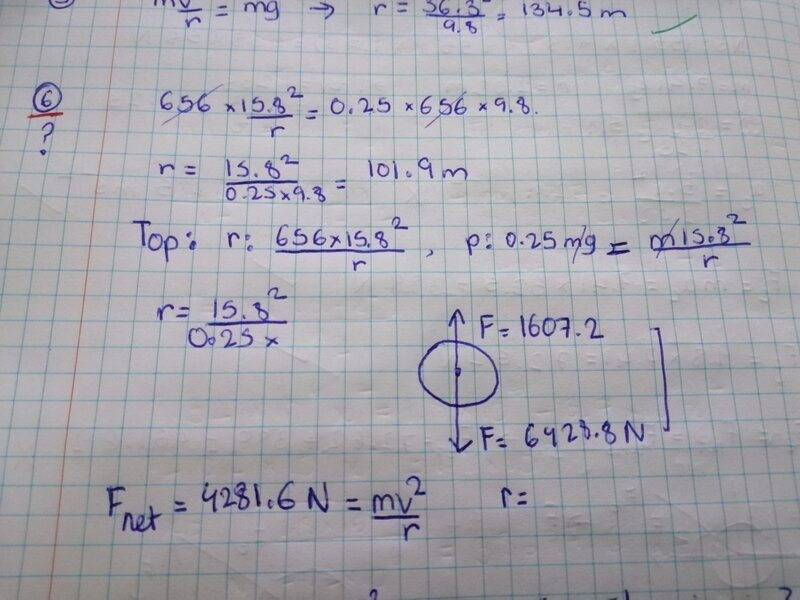# Circular motion problem

• bloodlust_X

#### bloodlust_X

Homework Statement
During the Physics Field Trip to Six Flags, Skyler and Jayson experienced a looping roller coaster ride for the first time. Their 656-kg roller coaster car was moving at 15.8 m/s at the top of the loop where occupants experienced a normal force equivalent to 1/4-th their weight. Determine the radius of curvature of the loop.
Relevant Equations
So I tried using F = mv^2/r, and f =mg to cancel mass but it didn't work, also its confusing which forces are supposed to be used?
Fnet would otherwise be 4281N, after subtracting 1/4 of rollercoaster's weight?

Please give us your actual attempt including the equations you wrote down. Show us what you did, don’t just describe it in words.

occupants experienced a normal force equivalent to 1/4-th their weight.

after subtracting 1/4 of rollercoaster's weight
It's the occupants that you have the normal force info on. Have you drawn a Free Body Diagram for one of those? What forces does it show?

Btw, you can't actually find the radius of the loop as a track, only the radius of arc the occupant's mass centre is following.

Please give us your actual attempt including the equations you wrote down. Show us what you did, don’t just describe it in words.It's the occupants that you have the normal force info on. Have you drawn a Free Body Diagram for one of those? What forces does it show?

Btw, you can't actually find the radius of the loop as a track, only the radius of arc the occupant's mass centre is following.
so nothing to do with the mass of the rollercoaster in the problem?

That doesn’t really tell us what you did. Please type it out with your arguments in between the equations. (See the homework guidelines)

As @Orodruin notes, posting that working doesn't tell us what principles you are using. But reverse engineering your first equation, it says centripetal force = gravitational force/4. Is that what the question says?